Updating search results...

# 15 Results

View
Selected filters:
• Trigonometry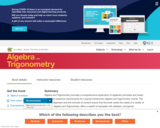Unrestricted Use
CC BY
Rating
0.0 stars

Algebra and Trigonometry provides a comprehensive exploration of algebraic principles and meets scope and sequence requirements for a typical introductory algebra and trigonometry course. The modular approach and the richness of content ensure that the book meets the needs of a variety of courses. Algebra and Trigonometry offers a wealth of examples with detailed, conceptual explanations, building a strong foundation in the material before asking students to apply what they’ve learned.

Subject:
Algebra
Mathematics
Trigonometry
Material Type:
Textbook
Author:
David Lippman
Jay Abramson
Jean-Marie Magnier
Melonie Rasmussen
Nicholas Belloit
Rachael Gross
Rick Norwood
Valeree Falduto
01/29/2015Conditional Remix & Share Permitted
CC BY-NC-SA
Rating
0.0 stars

This College Algebra text will cover a combination of classical algebra and analytic geometry, with an introduction to the transcendental exponential and logarithmic functions. If mathematics is the language of science, then algebra is the grammar of that language. Like grammar, algebra provides a structure to mathematical notation, in addition to its uses in problem solving and its ability to change the appearance of an expression without changing the value.

Subject:
Algebra
Mathematics
Trigonometry
Material Type:
Textbook
Author:
Richard W. Beveridge
08/13/2020Conditional Remix & Share Permitted
CC BY-NC-SA
Rating
0.0 stars

This book was designed as an introductory trigonometry textbook for college students with the explicit goal of reducing textbook costs.

Subject:
Algebra
Mathematics
Trigonometry
Material Type:
Textbook
Provider:
Stitz-Zeager Open Source Mathematics
Author:
Carl Stitz
Jeff Zeager
07/15/2011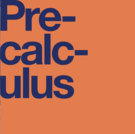Unrestricted Use
CC BY
Rating
0.0 stars

This OER is a list of Discussion Questions for a PreCalculus course using the OpenStax PreCalculus Textbook. There are eight discussion questions included in the Word document which has the equations better formatted.

Subject:
Algebra
Calculus
Higher Education
Mathematics
Trigonometry
Material Type:
Activity/Lab
Homework/Assignment
Author:
Larry Whittington
06/21/2022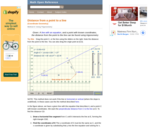Rating
0.0 stars

An interactive applet and associated web page that demonstrate how to find the perpendicular distance between a point and a line using trigonometry, given the coordinates of the point and the slope/intercept of the line. The applet has a line with sliders that adjust its slope and intercept, and a draggable point. As the line is altered or the point dragged, the distance is recalculated. The grid and coordinates can be turned on and off. The distance calculation can be turned off to permit class exercises and then turned back on the verify the answers. The applet can be printed as it appears on the screen to make handouts. The web page has a full description of the concept of the concepts, a worked example and has links to other pages relating to coordinate geometry. Applet can be enlarged to full screen size for use with a classroom projector. This resource is a component of the Math Open Reference Interactive Geometry textbook project at http://www.mathopenref.com.

Subject:
Geometry
Mathematics
Trigonometry
Material Type:
Simulation
Provider:
Math Open Reference
Author:
John Page
06/03/2021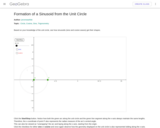Conditional Remix & Share Permitted
CC BY-SA
Rating
0.0 stars

Based on knowledge of the unit Circles, students see how sinusoids (Sine and coSine waves) get their shapes.

Subject:
Geometry
Mathematics
Trigonometry
Material Type:
Simulation
Provider:
GeoGebra
Provider Set:
GeoGebraTube
04/26/2012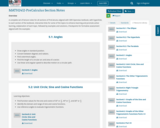Unrestricted Use
CC BY
Rating
0.0 stars

A complete set of lecture notes for all sections of PreCalculus aligned with OER Openstax textbook, with hyperlinks to each section of the textbook, interactive links for some of the topics to enhance learning and promote active learning, exlplanation of each topic, followed by examples and solutions, Checkpoints for formative assessment aligned with the examples

Subject:
Trigonometry
Material Type:
Lecture Notes
Author:
Shaghayegh Setayesh (She/Her/Hers)
Andras Balogh
Timothy Huber
03/04/2022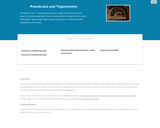Rating
0.0 stars

Precalculus 1 & 2 / Trigonometry provides a study of functions and their graphs, including polynomial, rational, exponential, and logarithmic functions. Additionally, right-triangle trigonometry, trigonometric functions and their applications are covered.

Subject:
Calculus
Mathematics
Trigonometry
Material Type:
Full Course
Textbook
Provider:
Lumen Learning
Provider Set:
Candela Courseware
Author:
David Lippman
Melonie Rasmussen
08/13/2020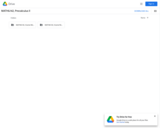Unrestricted Use
CC BY
Rating
0.0 stars

This course will cover families of trigonometric functions, their inverses, properties, graphs, and applications. Additionally we will study trigonometric equations and identities, the laws of sines and cosines, polar coordinates and graphs, parametric equations and elementary vector operations.Login: guest_oclPassword: ocl

Subject:
Mathematics
Trigonometry
Material Type:
Full Course
Homework/Assignment
Lecture Notes
Lesson Plan
Syllabus
Provider:
Washington State Board for Community & Technical Colleges
Provider Set:
Open Course Library
10/31/2011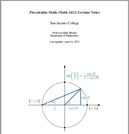Conditional Remix & Share Permitted
CC BY-NC-SA
Rating
0.0 stars

This is a focused collection of notes for a course in precalculus math taught at San Jacinto College (Houston, Texas). In less than 260 pages, the course offers a compact delivery of the core concepts that students ought to understand prior to taking calculus. In addition to learning algebraic and computational skills, the course is designed for learning how to think mathematically. The chapters on mathematical language and problem-solving highlight this latter objective.

Subject:
Calculus
Functions
Mathematics
Trigonometry
Material Type:
Full Course
Homework/Assignment
Lecture Notes
Textbook
Author:
Mark Moodie
07/12/2023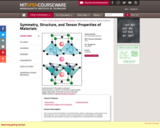Conditional Remix & Share Permitted
CC BY-NC-SA
Rating
0.0 stars

This course covers the derivation of symmetry theory; lattices, point groups, space groups, and their properties; use of symmetry in tensor representation of crystal properties, including anisotropy and representation surfaces; and applications to piezoelectricity and elasticity.

Subject:
Mathematics
Trigonometry
Material Type:
Full Course
Provider:
MIT
Provider Set:
MIT OpenCourseWare
Author:
Wuensch, Bernhardt
01/01/2005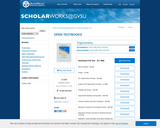Conditional Remix & Share Permitted
CC BY-NC-SA
Rating
0.0 stars

This trigonometry textbook is different than other trigonometry books in that it is free to download, and the reader is expected to do more than read the book and is expected to study the material in the book by working out examples rather than just reading about them. So this book is not just about mathematical content but is also about the process of learning and doing mathematics. That is, this book is designed not to be just casually read but rather to be engaged.

Since this can be a difficult task, there are several features of the book designed to assist students in this endeavor. In particular, most sections of the book start with a beginning activity that review prior mathematical work that is necessary for the new section or introduce new concepts and definitions that will be used later in that section. Each section also contains several progress checks that are short exercises or activities designed to help readers determine if they are understanding the material. In addition, the text contains links to several interactive Geogebra applets or worksheets. These applets are usually part of a beginning activity or a progress check and are intended to be used as part of the textbook.

The authors are very interested in constructive criticism of the textbook from the users of the book, especially students, who are using or have used the book. Please send any comments you have to trigtext@gmail.com.

Subject:
Mathematics
Trigonometry
Material Type:
Textbook
Provider:
Grand Valley State University
Author:
Steven Schlicker
Ted Sundstrom
12/01/2016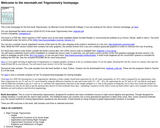Some Rights Reserved
Rating
0.0 stars

Book description: This is a text on elementary trigonometry, designed for students who have completed courses in high-school algebra and geometry. Though designed for college students, it could also be used in high schools. The traditional topics are covered, but a more geometrical approach is taken than usual. Also, some numerical methods (e.g. the secant method for solving trigonometric equations) are discussed. A brief tutorial on using Gnuplot to graph trigonometric functions is included.

There are 495 exercises in the book, with answers and hints to selected exercises.

Subject:
Mathematics
Trigonometry
Material Type:
Textbook
Provider:
Schoolcraft College
Author:
Michael Corral
08/13/2020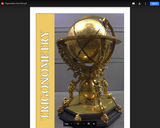Conditional Remix & Share Permitted
CC BY-NC-SA
Rating
0.0 stars

The precursors to what we study today as Trigonometry had their origin in ancient Mesopotamia, Greece and India. These cultures used the concepts of angles and lengths as an aid to understanding the movements of the heavenly bodies in the night sky. Ancient trigonometry typically used angles and triangles that were embedded in circles so that many of the calculations used were based on the lengths of chords within a circle. The relationships between the lengths of the chords and other lines drawn within a circle and the measure of the corresponding central angle represent the foundation of trigonometry - the relationship between angles and distances.

Subject:
Mathematics
Trigonometry
Material Type:
Textbook
Author:
Richard W. Beveridge
08/13/2020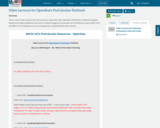Unrestricted Use
CC BY
Rating
0.0 stars

This is a list of video lectures that can be used in conjunction with OpenStax&#39;s PreCalculus Textbook that gives faculty and students addtional resources to utilize throughout the semester of a PreCalculus course. MATH 2412 and MATH 1313 PreCalculus courses among others may benefit from this resource.

Subject:
Algebra
Calculus
Functions
Trigonometry
Material Type:
Activity/Lab
Interactive
Lecture
Lesson
Teaching/Learning Strategy
Unit of Study
Author:
Larry Whittington